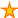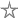# Calculating Elapsed Time

17 votes:45,414 views      1 comment
by Allen Browne, 20 April 2005    (for ALL VERSIONS of Access)

### Microsoft Access Tips for Casual Users

Provided by Allen Browne, November 2003.  Updated March 2007.

# Calculating elapsed time

How do you calculate the difference between two date/time fields, such as the hours worked between clock-on and clock-off?

Use DateDiff() to calculate the elapsed time. It returns whole numbers only, so if you want hours and fractions of an hour, you must work in minutes. If you want minutes and seconds, you must get the difference in seconds.

Let's assume a date/time field named StartDateTime to record when the employee clocks on, and another named EndDateTime for when the employee clocks off. To calculate the time worked, create a query into this table, and type this into the Field row of the query design grid:

`    Minutes: DateDiff("n", [StartDateTime], [EndDateTime])`

Minutes is the alias for the calculated field; you could use any name you like. You must use "n" for DateDiff() to return minutes: "m" returns months.

To display this value as hours and minutes on your report, use a text box with this Control Source:

`    =[Minutes] \ 60 & Format([Minutes] Mod 60, "\:00")`

This formula uses:

• the integer division operator (\) rather than regular division (/), for whole hours only;
• the Mod operator to get the left over minutes after dividing by 60;
• the Format() function to display the minutes as two digits with a literal colon.

Do not use the formula directly in the query if you wish to sum the time; the value it generates is just a piece of text.

If you need to calculate a difference in seconds, use "s":

`    Seconds: DateDiff("s", [StartDateTime], [EndDateTime])`

You can work in seconds for durations up to 67 years.

If you need to calculate the amount of pay due to the employee based on an HourlyRate field, use something like this:

`    PayAmount: Round(CCur(Nz(DateDiff("n", [StartDateTime], [EndDateTime]) * [HourlyRate] / 60, 0)), 2)`

The formula:

• calculates the minutes;
• multiples by the hourly rate;
• divides by 60 (minutes in an hour);
• converts a Null to zero (because currency cannot be Null);
• typecasts to Currency (the desired output, and for accurate rounding);
• and rounds the result to two decimal places (i.e. the nearest cent).

Note: Access 97 and earlier do not have the Round() function, but you can download one.

## Alternative functions

Doug Steele and Graham Seach have developed a more complete DateDiff. It shows the difference between two date/time values in days and hours and minutes and seconds.

To compare a date/time value to now, and return something readable such as "next week", or "in 5 hours", or "2 years ago", see Constructing Modern Time Elapsed Strings.

 Home Index of tips Top

Rate this article:This is a cached tutorial, reproduced with permission.